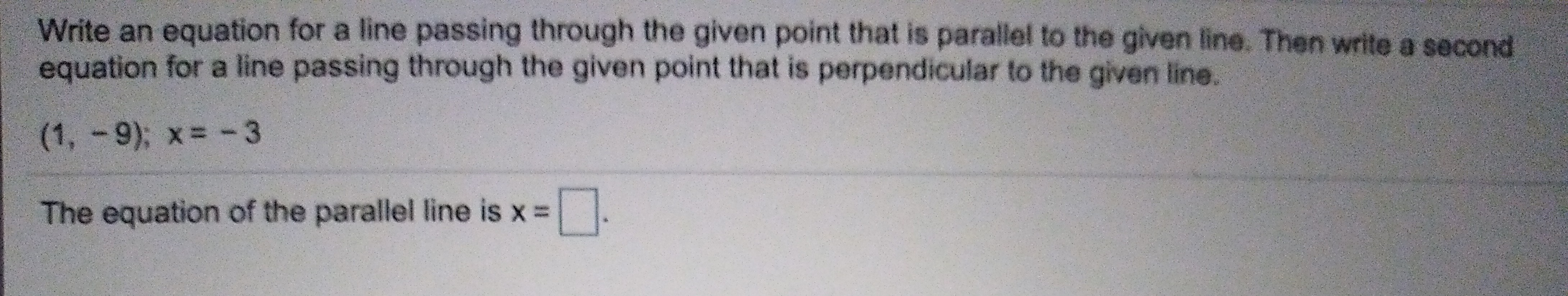# Write an equation for a line passing through the given point that is parallel to the given line. Then write a secondequation for a line passing through the given point that is perpendicular to the given line.(1, 9); x-3The equation of the parallel line is x= |

Question
1 viewshelp_outlineImage TranscriptioncloseWrite an equation for a line passing through the given point that is parallel to the given line. Then write a second equation for a line passing through the given point that is perpendicular to the given line. (1, 9); x-3 The equation of the parallel line is x= | fullscreen
check_circle

Step 1

Since the given equation is a vertical line, the slope of the vertical line is undefined.  That is, 0y = x + 3.

It is known that, the slopes of the parallel lines are the same. Thus, the slope of the line is undefined.

Thus, the slope of the equation for a line passing through the point (1, –9) parallel to the line x = –3 is undefined.

It is known that, the equation of the line passing through the point (a, b) and parallel to the vertical line is x = a.

Therefore, the equation of the line passing through the point (1, –9) parallel to the line x = –3 is x = 1.

Step 2

It is known that, if two lines are perpendicular, then the product of their slopes is equal to –1. T...

### Want to see the full answer?

See Solution

#### Want to see this answer and more?

Solutions are written by subject experts who are available 24/7. Questions are typically answered within 1 hour.*

See Solution
*Response times may vary by subject and question.
Tagged in

### Equations and In-equations